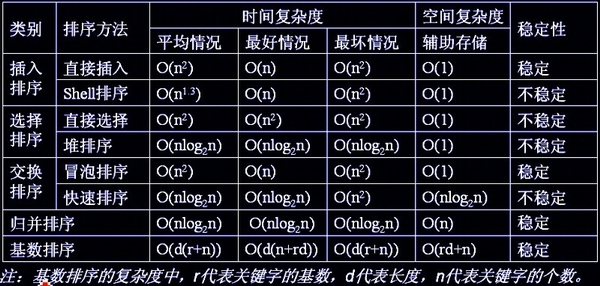# 【排序】八大排序算法简介及它们各自的特点总结

## 概述：

一般使用的八大排序算法是：插入排序、选择排序、冒泡排序、希尔排序、归并排序、快速排序、堆排序、基数排序，每个方法有其适合的使用场景，可以根据具体数据进行选择.

几个概念：

内部排序：排序期间元素全部存放在内存中的排序；

外部排序：排序期间元素无法全部存放在内存中，必须在排序过程中根据要求不断地进行内外存之间移动地排序；

(这八种排序算法中除了多路归并排序是外部排序，其他都是内部排序)

稳定性：指的是经过排序后，值相同的元素保持原来顺序中的相对位置不变.

各算法时间复杂度、空间复杂度、所需辅助空间与稳定性如下：## 1.冒泡排序：

void bubbleSort(vector<int> &s){
for (int i = 0; i < s.size(); i++){
for (int j = 0; j < s.size() - 1 - i; j++){
if (s[j] > s[j + 1])  swap(s[j], s[j + 1]);
}
}
}

## 2.插入排序：

void insertSort(vector<int> &s){
if (s.size() > 1){
for (int i = 1; i < s.size(); i++){
for (int j = i; j > 0 && s[j] < s[j - 1]; j--){
swap(s[j], s[j - 1]);
}
}
}
}

void binaryInsertSort(vector<int> &s){
int low, high, m, temp, i, j;
for (i = 1; i < s.size(); i++){
//采用折半查找方法，寻找应该插入的位置
low = 0;
high = i - 1;
while (low <= high){
m = (low + high) / 2;
if (s[m] > s[i])  high = m - 1;
else  low = m + 1;
}
//统一移动元素，将该元素插入到正确的位置
temp = s[i];
for (j = i; j > high + 1; j--){
s[j] = s[j - 1];
}
s[high + 1] = temp;
}
}

1.稳定;

2.最坏情况下比较n*(n-1)/2次，最好情况下比较n-1次;

3.第k次排序后，前k个元素已经是从小到大排好序的

## 3.简单选择排序：

void selectSort(vector<int> &s){
if(s.size()>1){
for(int i=0;i<s.size();i++){
int min=i;
for(int j=i+1;j<s.size();j++){
if(s[j]<s[min])  min=j;
}
swap(s[i], s[min]);
}
}
}

## 4.快速排序：

void quickSort(vector<int> &s, int low, int high){
if (low >= high)  return;
int l = low, r = high, val = s[low];
while (l < r){
while (l < r&&s[r] >= val)  r--;
if (l < r)  s[l++] = s[r];
while (l < r&&s[l] <= val)  l++;
if (l < r)  s[r--] = s[l];
}
s[l] = val;
quickSort(s, low, l - 1);
quickSort(s, r + 1, high);
}

1.不稳定；
2.快速排序过程中不会产生有序子序列，但每一趟排序后都有一个元素放在其最终位置上；
3.每次选择的关键值可以把数组分为两个子数组的时候，快速排序算法的速度最快，当数组已经是正序或逆序时速度最慢；
4.递归次数与每次划分后得到的分区的处理顺序无关；
5.对n个关键字进行快速排序，最大递归深度为n，最小递归深度为log2n；

//进行区域的划分
int partition(vector<int> &s, int low, int height){
int val = s[low];
while (low < height){
while (low < height&&s[height] >= val)
height--;
s[low] = s[height];
while (low < height&&s[low] <= val)
low++;
s[height] = s[low];
}
s[low] = val;
return low;
}
//排序
void quickSortNonRecursive(vector<int> &s, int low, int height){
stack<int> p;
if (low < height){
int mid = partition(s, low, height);
if (mid - 1 > low){
p.push(low);
p.push(mid - 1);
}
if (mid + 1 < height){
p.push(mid + 1);
p.push(height);
}
while (!p.empty()){
int qHeight = p.top();
p.pop();
int pLow = p.top();
p.pop();
int pqMid = partition(s, pLow, qHeight);
if (pqMid - 1 > pLow){
p.push(pLow);
p.push(pqMid - 1);
}
if (pqMid + 1 < qHeight){
p.push(pqMid + 1);
p.push(qHeight);
}
}
}
}

## 5.希尔排序：

void shellSort(vector<int> &s){
int n = s.size(), h = 1;
while (h < n / 3)  h = 3 * h + 1;
while (h >= 1){
for (int i = h; i < n; i++){
for (int j = i; j >= h && s[j] < s[j - h]; j -= h){
swap(s[j], s[j - h]);
}
}
h /= 3;
}
}

## 6.归并排序：

const int maxn=500000,INF=0x3f3f3f3f;
int L[maxn/2+2],R[maxn/2+2];
void merge(vector<int> &a,int n,int left,int mid,int right){
int n1=mid-left,n2=right-mid;
for(int i=0;i<n1;i++)  L[i]=a[left+i];
for(int i=0;i<n2;i++)  R[i]=a[mid+i];
L[n1]=R[n2]=INF;
int i=0,j=0;
for(int k=left;k<right;k++){
if(L[i]<=R[j])  a[k]=L[i++];
else  a[k]=R[j++];
}
}
void mergesort(vector<int> &a,int n,int left,int right){
if(left+1<right){
int mid=(left+right)/2;
mergesort(a,n,left,mid);
mergesort(a,n,mid,right);
merge(a,n,left,mid,right);
}
}

## 7.堆排序：

void max_heapify(vector<int> &arr, int start, int end) {
//建立父节点指标和子节点指标
int son = dad * 2 + 1;
while (son <= end) { //若子节点指标在范围内才做比较
if (son + 1 <= end && arr[son] < arr[son + 1]) //先比较两个子节点大小，选择最大的
son++;
return;
else { //否则交换父子内容再继续子节点和孙节点比较
son = dad * 2 + 1;
}
}
}

void heap_sort(vector<int> &arr, int len) {
//初始化，i从最後一个父节点开始调整
for (int i = len / 2 - 1; i >= 0; i--)
max_heapify(arr, i, len - 1);
//先将第一个元素和已经排好的元素前一位做交换，再从新调整(刚调整的元素之前的元素)，直到排序完毕
for (int i = len - 1; i > 0; i--) {
swap(arr, arr[i]);
max_heapify(arr, 0, i - 1);
}
}

## 8.基数排序：

//寻找数组中最大数的位数作为基数排序循环次数
int KeySize(vector<int> &s, int n){
int key = 1;
for (int i = 0; i < n; i++){
int temp = 1;
int r = 10;
while (s[i] / r > 0){
temp++;
r *= 10;
}
key = (temp > key) ? temp : key;
}
return key;
}

//基数排序
int key = KeySize(s, n);
int bucket = { 0 };
int order = { 0 };
for (int r = 1; key > 0; key--, r *= 10){
for (int i = 0; i < n; i++){
int lsd = (s[i] / r) % 10;
bucket[lsd][order[lsd]++] = s[i];
}
int k = 0;
for (int i = 0; i < 10; i++){
if (order[i] != 0){
for (int j = 0; j < order[i]; j++)
s[k++] = bucket[i][j];
}
order[i] = 0;
}
}
}

posted on 2019-07-01 17:00  nibolyoung  阅读(...)  评论(...编辑  收藏